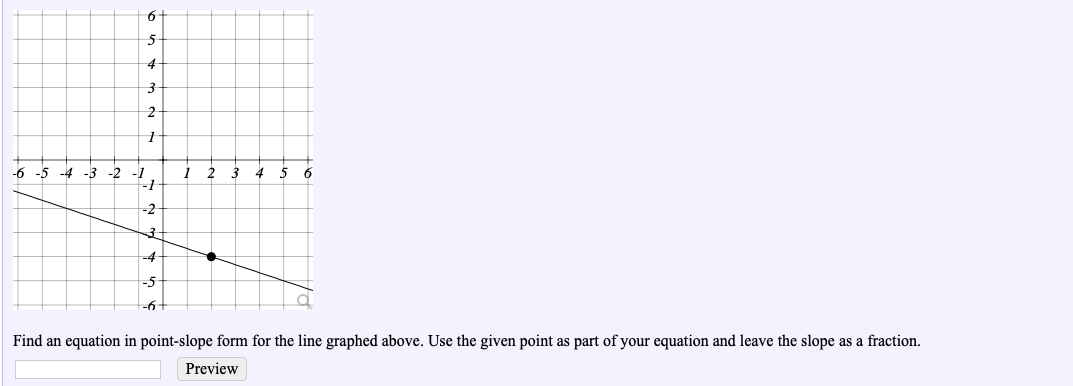# -6 Find an equation in point-slope form for the line graphed above. Use the given point as part of your equation and leave the slope as a fraction. Preview

Questionhelp_outlineImage Transcriptionclose-6 Find an equation in point-slope form for the line graphed above. Use the given point as part of your equation and leave the slope as a fraction. Preview fullscreen

### Want to see the step-by-step answer?